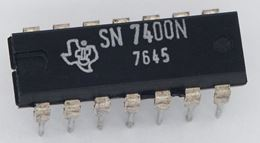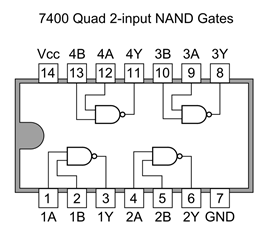# Logic Gates

Logic Gates process true and false values.

## And

The "and" logic gate must have both inputs true to become true.

Try clicking on the left hand lines here (green is true and blue is false):

images/gate.js?t=and&tchg=0&n=0&nchg=0

We can show that in a "truth table" (T is for true and F for false):

A B A and B F F F F T F T F F T T T

### Example: If we cut the grass and wash the car we get ice cream!

cut grass wash car ice cream F F F F T F T F F T T T

Only if we do both jobs do we get ice cream

## Or

The "or" logic gate can have either (or both) inputs true to become true:

images/gate.js?t=or&tchg=0&n=0&nchg=0

In a truth table:

A B A or B F F F F T T T F T T T T

### Example: If we cut the grass or wash the car we get ice cream!

cut grass wash car ice cream F F F F T T T F T T T T

In this case we can do either job (or both) to get ice cream. Let's wash the car.

## Xor (eXclusive Or)

Xor is like or except it becomes false when both inputs are true:

images/gate.js?t=xor&tchg=0&n=0&nchg=0

Here we see or and xor side-by-side:

A B A or B A xor B F F F F F T T T T F T T T T T F

Xor is like both your best friends fight. Life is fun with either one, but not both.

Think: "eXclusively yours" (no one else allowed).

## Not

We can also "not" or "invert":

images/gate.js?t=not&tchg=0&n=1&nchg=1
• not true is false
• not false is true

As a "truth table":

A not A F T T F

Important: it is the circle at the end that makes it "not":So we can "not" the other logic gates like this:

## Nand

Nand is "and" with a "not", so it is true except when both inputs are true:

images/gate.js?t=and&tchg=0&n=1&nchg=0

We can show that in a "truth table" (T is for true and F for false):

 A B A nand B A and B F F F T F T F T T F F T T T T F

## Nor

Nor is "or" and "not", so is false when either (or both) inputs are true:

images/gate.js?t=or&tchg=0&n=1&nchg=0

In a truth table:

 A B A nor B A or B F F F T F T T F T F T F T T T F

## Xnor

Xnor is xor with a not:

images/gate.js?t=xor&tchg=0&n=1&nchg=0

As a truth table:

A B A xnor B F F T F T F T F F T T T

Xnor is true when both inputs match (both true or both false).

## All Together Now

Here they are together:

A B and nand or nor xor xnor input output F F F T F T F T F T F T T F T F T F F T T F T F T T T F T F F T

See them in action here (try clicking on the name, or the output line):

images/gate.js?t=and&tchg=1&n=0&nchg=1

## In the Real World

This classic chip has 4 nand gates on it:It's circuit diagram looks like this:Example: you can connect pins 1 and 2 to different inputs, and will get an output from pin 3 except when both pins 1 and 2 are on.

(Note: power supply of 5 volts between "Vcc" and "GND")

There are many variations of these chips with different logic gates inside them. They operate really fast (millions of times a second), use very little power and cost very little. You can combine them to do amazing things.